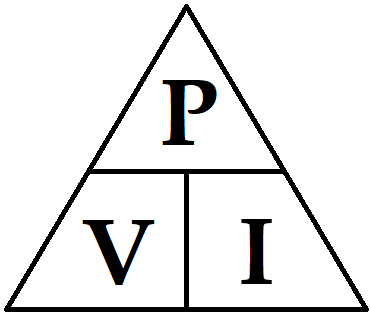# Amps to Watts Calculator

Enter Current and voltage values to calculate the Power in Watts.

You can also enter any two known values to calculate the third one, this way you can use it to convert Ampere to watts or voltage to watts or can also use it to convert Watts to voltage or watts to Ampere.

 Voltage (V): Volt Current (I) Amps Milliamps Power (P) Watts

This calculator will help you in finding the value of the Power (in watts) of the circuit by using Voltage and Current value. The Calculator works on the Watt’s Law formula given below:

```Power (P) = Voltage (V) x Current (I)
```

### Watt’s Law

According to the Watt’s Law the total power of the circuit is equal to the product of the current and voltage of the circuit. By using this formula you can even calculate the voltage and current if you have the other two values. The formulas follow the Watts’s law triangle,Where, P represent Power, V represents Voltage and I represents Current.

```Power (P) = Voltage (V) x Current (I)
Voltage (V) = Power (P) ÷ Current (I)
Current (I) = Power (P) ÷ Voltage (V)```

As, we know this calculator calculates the Power in Watts using the current and voltage value. What if you don’t have one of them or you have the resistance value of the circuit. For theoretical calculation you can use the below mentioned formulas:

```Power = (Voltage) 2 ÷ Resistance
Power = (Current) 2 x Resistance```